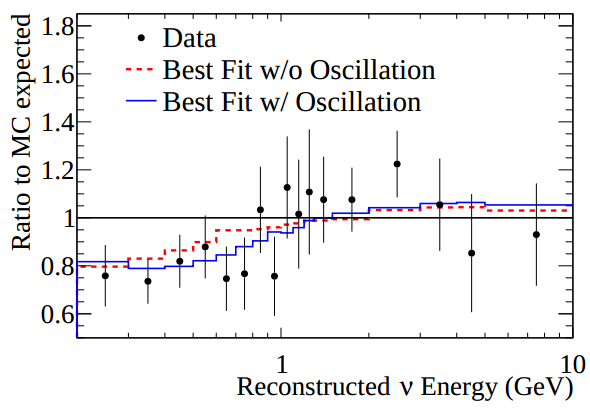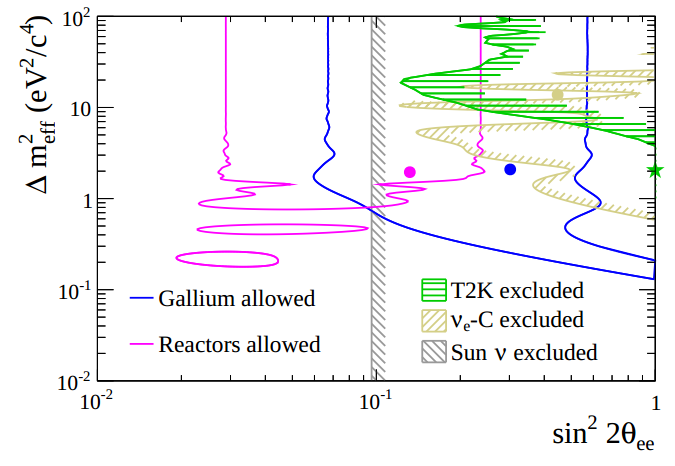# Paper of the month:  Search for short baseline νe disappearance with the T2K near detectorNeutrino oscillations have been confirmed in many experiments in the last two decades. Almost all the observed oscillations fit quite well in the standard three neutrino picture where we have electron, tau and muon ”type or flavour” neutrinos. Oscillations can occur as the flavour of the neutrino does not correspond to a distinct massive particle. The distinct mass-state neutrinos are the physical particles that move freely through space and are made up of a combination of electron, tau and muon flavour neutrinos. So if you observe a neutrino in flight, you might observe the muon component one time, and the electron component the next. It is this change of neutrino flavour over time that we call neutrino oscillations.

#### December 16, 2014 by tiinatimonen

The physics describing these oscillation are fully explained with six measurable  parameters.  Some of these  parameters are  measured  by the  T2K experiment in Japan. Among these  six parameters, there  are two squared mass differences (which represent how heavy each mass state  is in respect to the others),  three angles (which correspond to how much of each flavour is in each mass state)  and one CP violating phase.  However, not all experiments agree.  There are two experiments,  SAGE and GALLEX, which have seen a substantial deficit of electron neutrinos  originating  from intense radioactive sources at energies much larger than  the standard neutrino  mass differences. These anomalies can be accommodated  by expanding  the standard picture by one sterile neutrino,  i.e. expanding the parameter space by one more mass difference and three more mixing angles, one for each active neutrino  flavour. These new particles  are referred to as sterile neutrinos,  as they cannot  have weak interactions as the standard ”active” neutrinos  or we would have dis- covered them already.

In this paper, new data from the near detector of T2K experiment are used to analyse the νe  spectra  in the framework of an additional  sterile neutrino. It  has to be noted  that  other  anomalies  of neutrino  oscillations  have been seen in the  LSND and  MiniBOONE  experiments,  as well as in the  reactor experiments,  but these anomalies can not be explained by adding one sterile neutrino  because of the tension between appearance  and disappearance data. In the absence of a consensus candidate  model, the authors  believe that  an analysis  of the  most  simple  3+1  extension  can  give some insights of the existing anomalies.Figure 1: Passage of the muon neutrino beam from J-PARC to Super K

T2K  (Tokai  to  Kamioka)  is a long baseline  experiment,  with  the  goal to  measure  the  neutrino  oscillations  parameters.  The  pions  are  produced in the collisions of the proton  beam with a carbon target,  and they quickly decay to muons and muon neutrinos.  At the end of decay pipe, muons, kaons and other heavy particles are stopped  by a second layer of graphite,  but the neutrinos  pass through  this  layer.  The intensity  and direction  of the beam are controlled  by the  Interactive Neutrino  GRID  (INGRID)  near  detector, which is placed at  280 m away from the source, in the center  of the beam. Another  detector,  ND280 is also placed  at  280 m,  but  2.5 degrees off the neutrino  beam  axis.   The  further  detector,  Super  K, which is the primary physics detector,  is at  275 km, also 2.5 degrees of axis.  The angle is set to peak the  neutrino  energy around  500 MeV. The  near  detector  ND280 can look for the existence of a heavier sterile neutrino.   The result  presented  in the paper are based on data  taken  from January 2010 to May 2013.

A standard procedure  that  scientists  use for determination of the  un- known parameters, as well as seeing if a given theory  fits well with observational data is called ”minimizing the χ2 function”.  The χ2 function describes disagreements  between real data  and the predicted  ones.  If you take a theory and compare its predictions  to that  of an experiment,  if the theory  and experiment agree the χ2 is a small number,  but  if the theory  predicts  a lot of events that  are not observed, then the associated χ2 is large.  The best fit value is obtained  by looking for the  smallest  value of the  χ2, hence ”mini-mizing the χ2 ”, as this by definition is the point which the theory  and the experiment agree best.

If you look at  Figure 2, we see the black dots representing  the data  ob- served in the T2K detector.  The black bars represent the ”errors” or ”uncertainties”  in the measurements. As we can see the blue line representing  how well a theory with and extra sterile neutrino ts the data better than the red dashed line which does not have any extra particles or physics at work. The best t occurs for maximal mixing and mass di erence of 2:05 eVIn this analysis mixing of the sterile neutrino with muon neutrino is set to 0, but the exclusion curve does not depend much on this value, as long as it is in the region allowed by other experiments.Figure 2: Ratio of the predicted over measured data for both oscillations with the parameters value obtained from the fit (solid blue), and non-oscillation case (red dashed line). Systematic and statistical errors are also shown on the plot as the vertical black bars on each data point.

However, this does not mean we can declare we have discovered a new particle! If you look again at the data in Figure 2, although the blue curve does i t better, the red curve is not a bad t itself. In fact you could easily believe that the red curve ts ne, with one or two points that are statistically a bit low due to random chance. To clarify not just if it ts better, but how signi cant is the t, physicists use a concept called a p-value of a hypothesis. This answers the question how likely is it that we would get such data if a hypothesis is actually true, taking into account random uctuations. So we can ask what is the p-value of the no-oscillation hypothesis (i.e. what is the probability of obtaining the observed data (or a more extreme result) when there is no extra sterile). The value obtained by T2K in this analysis is 0.085 or 8.5%, and therefore not statistically signi cant to reject the null hypothesis.

Although the results from T2K near detector may point to the existence of a sterile neutrino, we require more than one experiment, and at a much higher signi cance to declare a discovery. This concept of multiple experiments showing a signal is very important in physics, as it prevents accidental claims before we have sucient evidence to back them up. Figure 3 shows the allowed parameter space by the reactor and Gallium anomalies as well as the T2K best t by a green star. More data will reduce the statistical uncertainty and could allow T2K to improve the short baseline data sterile neutrino analyses.Figure 3: Summary of the exclusion curves obtained from T2K and other experiments available in the literature. The allowed regions from gallium and reactor anomalies are also illustrated.

Text by Marija Kekic (IFIC) and Mark Ross-Lonergan (IPPP)